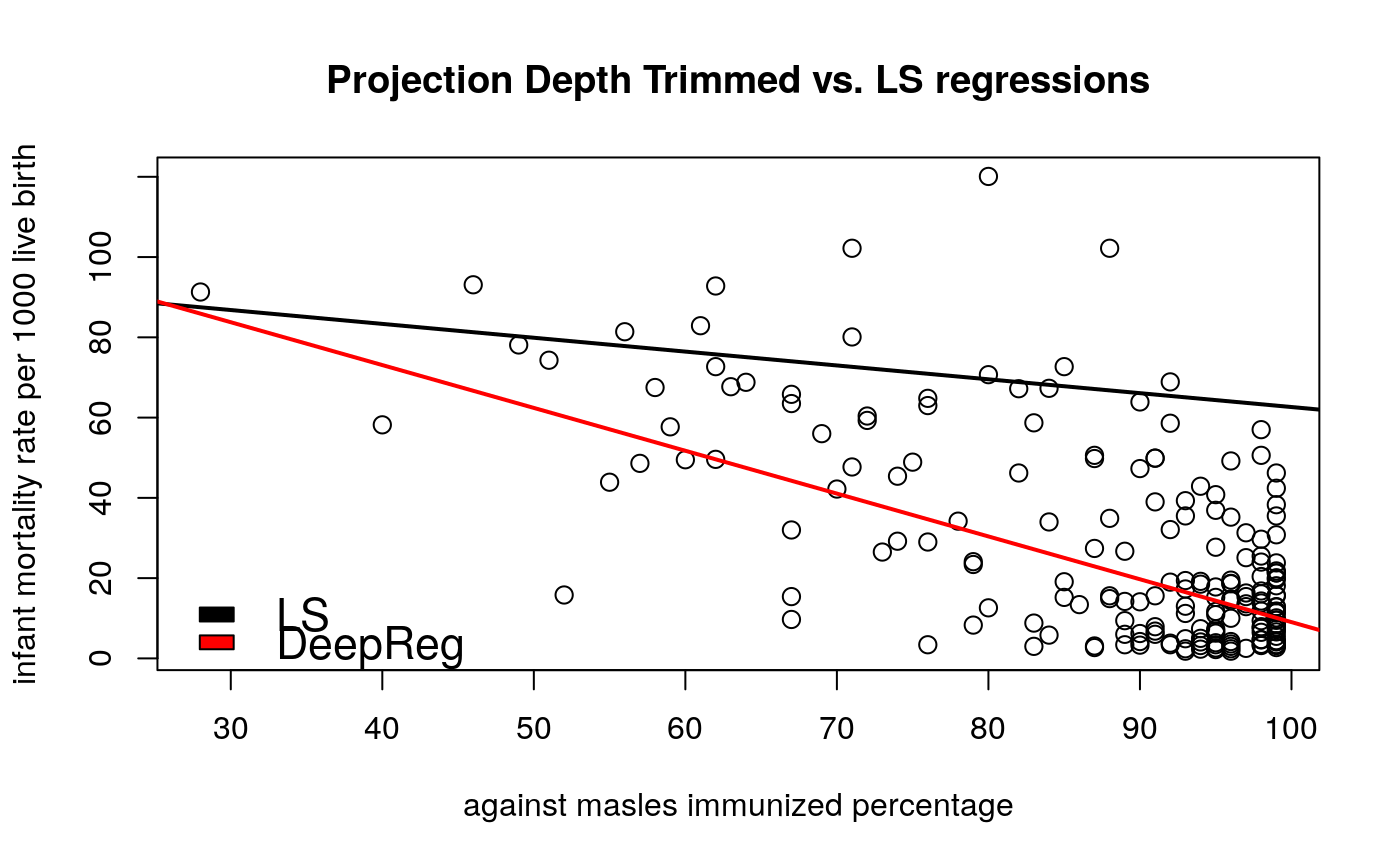This function calculates deepest regression estimator for simple regression.

deepReg2d(x, y)

## Arguments

x Independent variable. Dependent variable.

## Details

Function originates from an original algorithm proposed by Rousseeuw and Hubert. Let $${Z} ^ {n} = {(x_1, y_1), ..., (x_n, y_n)} \subset {{R} ^ {d}}$$ denotes a sample considered from a following semiparametric model: $${{y}_{l}} = {{a}_{0}} + {{a}_{1}}{{x}_{1l}} + ... + {{a}_{(d - 1)l}}{{x}_{(d - 1)l}} + {{\varepsilon }_{l}}, {l = 1, ..., n}$$, we calculate a depth of a fit $$\alpha = (a_{0}, ..., a_{d - 1})$$ as $$RD(\alpha, {{Z} ^ {n}}) = {u \ne 0}\min\sharp {l: \frac{ {{r}_{l}}(\alpha) }{ {{u} ^ {T}}{{x}_{l}} } < 0, l = 1, ..., n}$$, where $$r(\cdot)$$ denotes the regression residual, $$\alpha = (a_{0}, ..., a_{d - 1})$$, $${u} ^ {T}{x}_{l} \ne 0$$. The deepest regression estimator $$DR(\alpha, {{Z} ^ {n}})$$ is defined as $$DR(\alpha, {{Z} ^ {n}}) = {\alpha \ne 0}\,\arg\max\,RD(\alpha, {{Z} ^ {n}})$$

Rousseeuw J.P., Hubert M. (1998), Regression Depth, Journal of The American Statistical Association, vol.94.

## Examples


# EXAMPLE 1
data(pension)
plot(pension)abline(
lm(Reserves ~ Income, data = pension),
lty = 3,
lwd = 2) # lmabline(
deepReg2d(pension[, 1], pension[, 2]),
lwd = 2) # deepreg2d# EXAMPLE 2
data(under5.mort)
data(inf.mort)
data(maesles.imm)
data2011 <- na.omit(
cbind(under5.mort[, 22], inf.mort[, 22],
maesles.imm[, 22]))

x <- data2011[, 3]
y <- data2011[, 2]
plot(
x, y,
cex = 1.2,
ylab = "infant mortality rate per 1000 live birth",
xlab = "against masles immunized percentage",
main = "Projection Depth Trimmed vs. LS regressions"
)abline(lm(x ~ y), lwd = 2, col = "black") # lmabline(
deepReg2d (x, y),
lwd = 2, col = "red"
) # trimmed reg
legend(
"bottomleft",
c("LS", "DeepReg"),
fill = c("black", "red"),
cex = 1.4,
bty = "n"
)